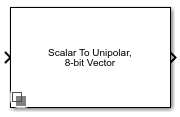# Binary Vector Conversion

Convert scalar integer to binary logic vector and vice versa

• Library:
• Mixed-Signal Blockset / Utilities

•## Description

The Binary Vector Conversion block encodes, decodes, and manipulates binary coded vectors. You can convert a scalar input to a logical (Boolean) vector signal using binary magnitude or two's complement and vice versa. You can also resize and reverse the bit index arrangement of the binary logic vectors.

## Ports

### Input

expand all

Input signal, specified as a scalar or logical vector.

• When encoding, the input signal is a scalar.

• When decoding, the input signal is a logical (Boolean) vector.

Note

When converting to a scalar value from a bit stream, any input greater than 1 is considered as logical '1'. Similarly any input bit is less than 0 is considered logical '0'.

Data Types: `single` | `double` | `uint8` | `uint16` | `uint32` | `Boolean` | `fixdt(0,16)`

### Output

expand all

Output signal, specified as a scalar or logical vector.

• When encoding, the output signal is a logical (Boolean) vector.

• When decoding, the output signal is a scalar.

Data Types: `single` | `double` | `uint8` | `uint16` | `uint32` | `Boolean` | `fixdt(0,16)`

## Parameters

expand all

Type of conversion, specified as one of these:

• `Scalar to binary-coded vector` — Encode a scalar input signal to logical vector.

• `Binary-coded vector to scalar` — Decode a logical vector signal to scalar.

• `Resize binary-coded vector` — Resize a logical vector while preserving the sign using two's complement method.

• `Reverse binary-coded vector` — Reverse the bit index arrangement of a logical vector.

Note

When converting to a scalar value from a bit stream we will consider any input greater than 1 as a logical '1'. Similarly if the input bit is less than 0 (i.e. any -ve number) then it will be considered logical '0'.

#### Programmatic Use

 Block parameter: `Convert` Type: character vector Values: `Scalar to binary-coded vector`| ```Binary-coded vector to scalar```| ```Resize binary-coded vector```| ```Reverse binary-coded vector``` Default: `Scalar to binary-coded vector`

Method of encoding logical vector, specified as either ```Unipolar (magnitude)``` or ```Bipolar (2's complement)```.

#### Dependencies

To enable this parameter, set Convert to ```Scalar to binary-coded vector```, ```Binary-coded vector to scalar```, or ```Resize binary-coded vector```.

#### Programmatic Use

 Block parameter: `Encoding` Type: character vector Values: `Unipolar (magnitude)`| ```Bipolar (2's complement)``` Default: `Unipolar (magnitude)`

Number of vector elements in the input vector, specified as a positive real scalar.

By default, the least significant bit (LSB) is the first element of the vector (index 1). But you can also set the most significant bit (MSB) as the first element of the vector.

#### Dependencies

To enable this parameter, set Convert to `Binary-coded vector to scalar`, ```Resize binary-coded vector```, or ```Reverse binary-coded vector```.

#### Programmatic Use

 Block parameter: `InputLength` Type: character vector Values: positive real scalar Default: `8`

Number of vector elements in the output vector, specified as a positive real scalar.

By default, the least significant bit (LSB) is the first element of the vector (index 1). But you can also set the most significant bit (MSB) as the first element of the vector.

#### Dependencies

To enable this parameter, set Convert to ```Scalar to binary-coded vector``` or ```Resize binary-coded vector```.

#### Programmatic Use

 Block parameter: `OutputLength` Type: character vector Values: positive real scalar Default: `8`

Datatype of the output scalar. You can choose to inherit the datatype, specify directly, or express as a datatype object.

#### Dependencies

To enable this parameter, set Convert to `Binary-coded vector to scalar`.

#### Programmatic Use

 Block parameter: `DataType` Type: character vector Values: `Inherit: Inherit via back propagation`| `double`| `single`| `unit8`| `unit16`| `unit32`| `fixdt(0,16)` Default: `double`

## Version History

Introduced in R2021a